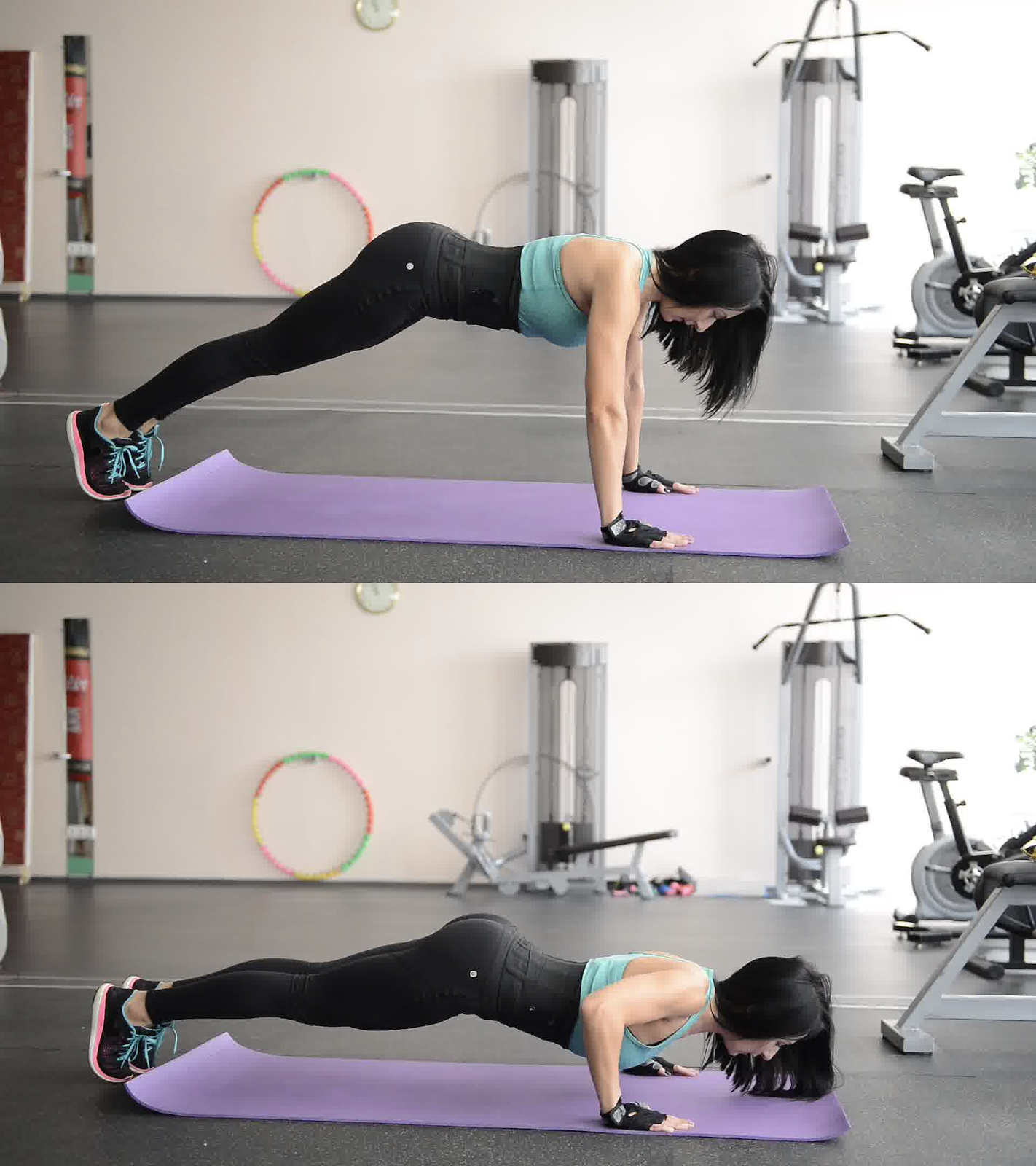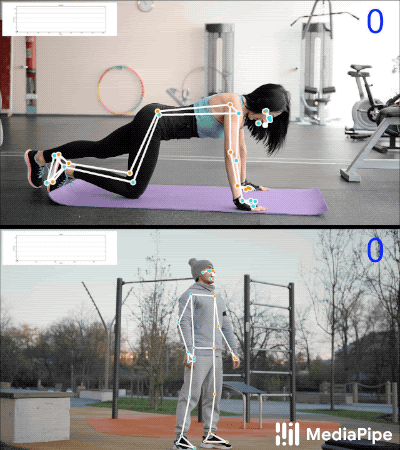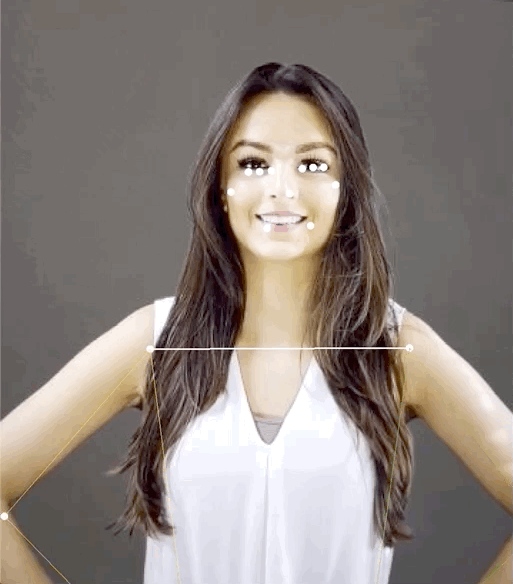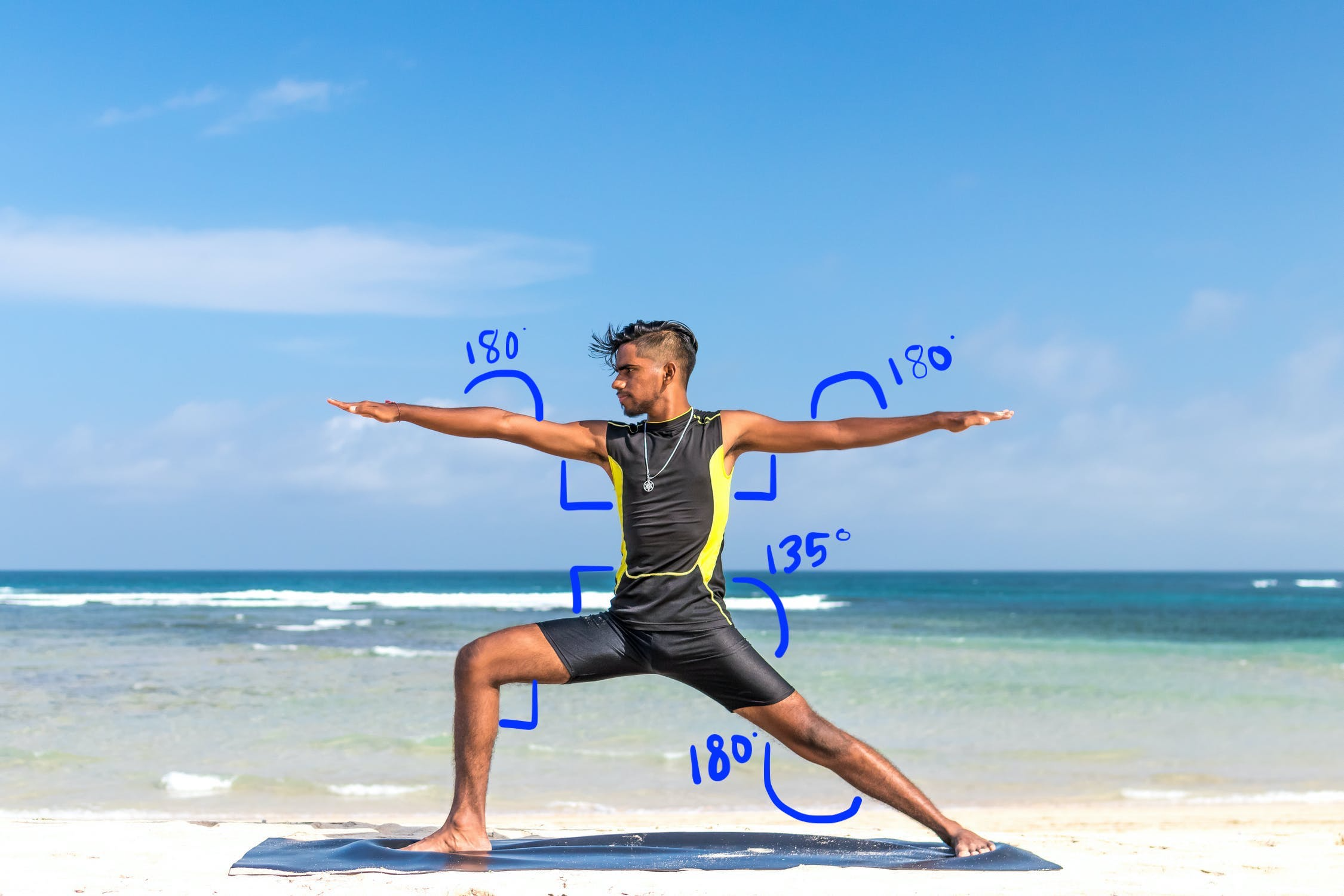# 姿勢分類選項

## 使用 k-NN 演算法計算姿勢分類與重複計數

### 1. 收集圖片樣本### 3. 訓練模型及計算重複次數

• 當「下移」姿勢首次傳遞指定的門檻時，演算法會標示「向下」姿勢輸入。
• 當機率降至門檻以下時，演算法會標示「向下」pose 已離開，並增加計數器。### 4. 與 ML Kit 快速入門導覽課程應用程式整合

#### 新增自己的 CSV 檔案

• 將 CSV 檔案加入應用程式的素材資源資料夾。
• PoseClassifierProcessor 中，更新 `POSE_SAMPLES_FILE``POSE_CLASSES` 變數，以符合您的 CSV 檔案和姿勢範例。
• 建構並執行應用程式。

## 計算地標距離，辨識簡單的手勢## 以角度經驗法則稱作瑜珈姿勢• 兩個角度的 90 度角
• 雙肘 180 度
• 前腿和腰部有 90 度角
• 膝蓋的 180 度角
• 腰圍為 135 度角

Once you've computed all the angles needed to identify the pose, you can check to see if there's a match, in which case you've recognized the pose.

### Kotlin

```fun getAngle(firstPoint: PoseLandmark, midPoint: PoseLandmark, lastPoint: PoseLandmark): Double {
var result = Math.toDegrees(atan2(lastPoint.getPosition().y - midPoint.getPosition().y,
lastPoint.getPosition().x - midPoint.getPosition().x)
- atan2(firstPoint.getPosition().y - midPoint.getPosition().y,
firstPoint.getPosition().x - midPoint.getPosition().x))
result = Math.abs(result) // Angle should never be negative
if (result > 180) {
result = 360.0 - result // Always get the acute representation of the angle
}
return result
}
```

### Java

```static double getAngle(PoseLandmark firstPoint, PoseLandmark midPoint, PoseLandmark lastPoint) {
double result =
Math.toDegrees(
atan2(lastPoint.getPosition().y - midPoint.getPosition().y,
lastPoint.getPosition().x - midPoint.getPosition().x)
- atan2(firstPoint.getPosition().y - midPoint.getPosition().y,
firstPoint.getPosition().x - midPoint.getPosition().x));
result = Math.abs(result); // Angle should never be negative
if (result > 180) {
result = (360.0 - result); // Always get the acute representation of the angle
}
return result;
}
```

### Kotlin

```val rightHipAngle = getAngle(
pose.getPoseLandmark(PoseLandmark.Type.RIGHT_SHOULDER),
pose.getPoseLandmark(PoseLandmark.Type.RIGHT_HIP),
pose.getPoseLandmark(PoseLandmark.Type.RIGHT_KNEE))
```

### Java

```double rightHipAngle = getAngle(
pose.getPoseLandmark(PoseLandmark.Type.RIGHT_SHOULDER),
pose.getPoseLandmark(PoseLandmark.Type.RIGHT_HIP),
pose.getPoseLandmark(PoseLandmark.Type.RIGHT_KNEE));
```

### Swift

```func angle(
firstLandmark: PoseLandmark,
midLandmark: PoseLandmark,
lastLandmark: PoseLandmark
) -> CGFloat {
atan2(lastLandmark.position.y - midLandmark.position.y,
lastLandmark.position.x - midLandmark.position.x) -
atan2(firstLandmark.position.y - midLandmark.position.y,
firstLandmark.position.x - midLandmark.position.x)
var degrees = radians * 180.0 / .pi
degrees = abs(degrees) // Angle should never be negative
if degrees > 180.0 {
degrees = 360.0 - degrees // Always get the acute representation of the angle
}
return degrees
}
```

### Objective-C

```(CGFloat)angleFromFirstLandmark:(MLKPoseLandmark *)firstLandmark
midLandmark:(MLKPoseLandmark *)midLandmark
lastLandmark:(MLKPoseLandmark *)lastLandmark {
CGFloat radians = atan2(lastLandmark.position.y - midLandmark.position.y,
lastLandmark.position.x - midLandmark.position.x) -
atan2(firstLandmark.position.y - midLandmark.position.y,
firstLandmark.position.x - midLandmark.position.x);
CGFloat degrees = radians * 180.0 / M_PI;
degrees = fabs(degrees); // Angle should never be negative
if (degrees > 180.0) {
degrees = 360.0 - degrees; // Always get the acute representation of the angle
}
return degrees;
}
```

### Swift

```let rightHipAngle = angle(
firstLandmark: pose.landmark(ofType: .rightShoulder),
midLandmark: pose.landmark(ofType: .rightHip),
lastLandmark: pose.landmark(ofType: .rightKnee))
```

### Objective-C

```CGFloat rightHipAngle =
[self angleFromFirstLandmark:[pose landmarkOfType:MLKPoseLandmarkTypeRightShoulder]
midLandmark:[pose landmarkOfType:MLKPoseLandmarkTypeRightHip]
lastLandmark:[pose landmarkOfType:MLKPoseLandmarkTypeRightKnee]];
```

[]
[]